## Friction Questions and Answers Part-4

1.A motorcyclist of mass m is to negotiate a curve of radius r with a speed v. The minimum value of the coefficient of friction so that this negotiation may take place safely, is
a) $v^{2} rg$
b) $\frac{v^{2}}{gr}$
c) $\frac{gr}{v^{2}}$
d) $\frac{g}{v^{2}r}$

Explanation: $\frac{v^{2}}{gr}$

2.On a rough horizontal surface, a body of mass 2 kg is given a velocity of 10 m/s. If the coefficient of friction is 0.2 and $g=10 m\diagup s^{2}$ , the body will stop after covering a distance of
a) 10 m
b) 25 m
c) 50 m
d) 250 m

Explanation: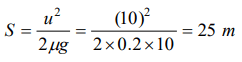3. A block of mass 50 kg can slide on a rough horizontal surface. The coefficient of friction between the block and the surface is 0.6. The least force of pull acting at an angle of 30° to the upward drawn vertical which causes the block to just slide is
a) 29.43 N
b) 219.6 N
c) 21.96 N
d) 294.3 N

Explanation: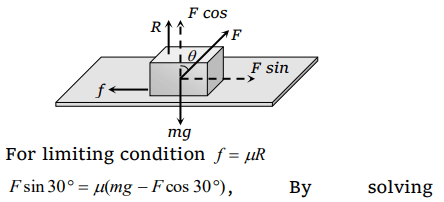F = 294.3 N

4.A body of 10 kg is acted by a force of 129.4 N if $g=9.8 m\diagup s^{2}$  . The acceleration of the block is $10 m\diagup s^{2}$  . What is the coefficient of kinetic friction
a) 0.03
b) 0.01
c) 0.30
d) 0.25

Explanation: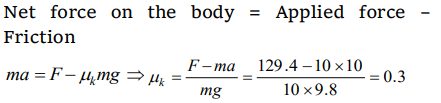5. Assuming the coefficient of friction between the road and tyres of a car to be 0.5, the maximum speed with which the car can move round a curve of 40.0 m radius without slipping, if the road is unbanked, should be
a) 25 m/s
b) 19 m/s
c) 14 m/s
d) 11 m/s

Explanation: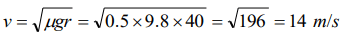6. Consider a car moving along a straight horizontal road with a speed of 72 km/h. If the coefficient of kinetic friction between the tyres and the road is 0.5, the shortest distance in which the car can be stopped is [ g = 10 ms-2]
a) 30 m
b) 40 m
c) 72 m
d) 20 m

Explanation: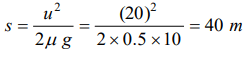7. A 500 kg horse pulls a cart of mass 1500 kg along a level road with an acceleration of $1 m\ s^{-2}$ . If the coefficient of sliding friction is 0.2, then the force exerted by the horse in forward direction is
a) 3000 N
b) 4000 N
c) 5000 N
d) 6000 N

Explanation:8. The maximum speed of a car on a road turn of radius 30m; if the coefficient of friction between the tyres and the road is 0.4; will be
a) 9.84 m/s
b) 10.84 m/s
c) 7.84 m/s
d) 5.84 m/s

Explanation: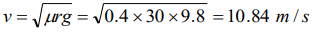9.A block of mass 50 kg slides over a horizontal distance of 1 m. If the coefficient of friction between their surfaces is 0.2, then work done against friction is
a) 98 J
b) 72J
c) 56 J
d) 34 J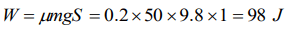10. On the horizontal surface of a truck $\left(\mu =0.6\right)$  , a block of mass 1 kg is placed. If the truck is accelerating at the rate of 5 m/sec2 then frictional force on the block will be